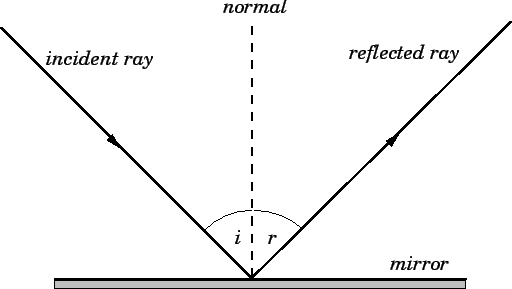# State the two laws of reflection of light

State the two laws of reflection of light.

The two laws of reflection can be stated as below:

1. The angle of incidence is equal to the angle of reflection.
2. The incident ray, the reflected ray and the normal to the mirror all lie in the same plane.

Laws of reflection:

1. Incident ray, the reflected ray, and the normal to the surface of the mirror all lie in the same plane.

2. The angle of reflection is equal to the angle of incidence . Both angles are measured with respect to the normal to the mirror